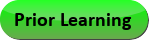Feb 05, 2023
2021-2022 College Catalog
 Select a Catalog 2022-2023 College Catalog 2021-2022 College Catalog [ARCHIVED CATALOG] 2020-2021 College Catalog [ARCHIVED CATALOG] 2019-2020 College Catalog [ARCHIVED CATALOG] DRAFT 2023-2024 College Catalog DRAFT [ARCHIVED CATALOG]
 HELP 2021-2022 College Catalog [ARCHIVED CATALOG] Print-Friendly Page (opens a new window)

# GTM 105 - Applied Technical Mathematics

3 Credits, 3 Contact Hours
3 lecture periods 0 lab periods

Applied geometry and trigonometry. Includes a brief review of operations with integers; review of pre-algebra; systems of measurement; solving problems involving right- and oblique triangle trigonometry, perimeter, areas, surface areas, and volumes; and practical applications.

Prerequisite(s): Within the last three years: With a grade of C or better: ICS 081  or completion of module 15 in MAT 089A  or satisfactory score on the Mathematics assessment test.
Gen-Ed: Meets CTE - M&S.Course Learning Outcomes
1. Use ratios and proportions to solve application problems involving similar shapes, percentages, and conversion of measures between metric and US customary systems..
2.  Solve application problems using algebraic techniques such as solving linear equations and manipulating and evaluating formulas.
3. Apply angle measurement, right- and oblique-triangle trigonometry to solve application problems.
4.  Calculate area and perimeter of polygons and circles, and volumes and surface areas of basic three-dimensional figures to solve application problems.
5. Apply concepts and techniques to practical math problems related to their specific subject areas

Outline:
I. Operations with Real Numbers

A. Integer numbers

B. Fractions

C. Decimals Powers and square roots

D. Ratios, proportions, ratios and percents

E. Dimensional analysis (includes Metric/US customary conversions) Order of operations

F.  Measurement

1.Lengths and angles

II. Equations and Formulas

A. One- and two-step equations

B. Formulas

C. Application problems

III. Elements of Geometry

A. Angles

1. Practical application (e.g., perpendicularity)

2. Complement/supplement

3. Conversion (decimal degrees/degrees-minutes-seconds)

IV. Triangles and Circles

A.  Triangles

1.  Types

2.  Right triangle and Pythagorean Theorem

3.  Congruent and similar triangles

4.  Oblique triangles

B.  Circles

1.  Identifying parts

2.  Circle measurements

3.  Area

III.  Trigonometry

A.  Right triangles

1.  Sine, cosine, and tangent

2.  Determining angles and sides

3.  Area and perimeter

VI. Plane Geometry

A.  Perimeter of polygons and circles

B.  Area of polygons and circles

V.  Solids

A.  Surface area of solids

B.  Volume of solids

VI. Program Specific Topics

A. Selected topics differentiated by programs (e.g., Cartesian plane and transformations, units of pressure, and Ohm’s Law).

Effective Term: